# 3. A silicon step junction has uniform impurity doping concentrations of N. 5 x 1015 cm-3 and Nd ...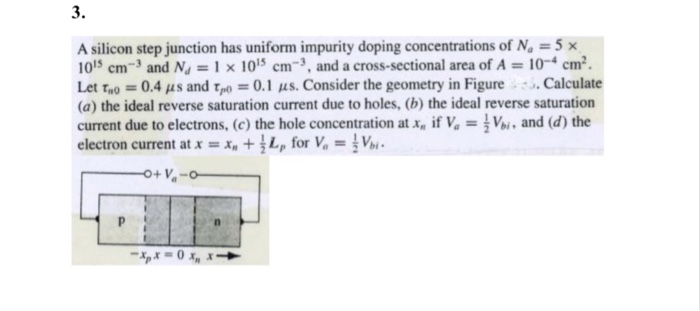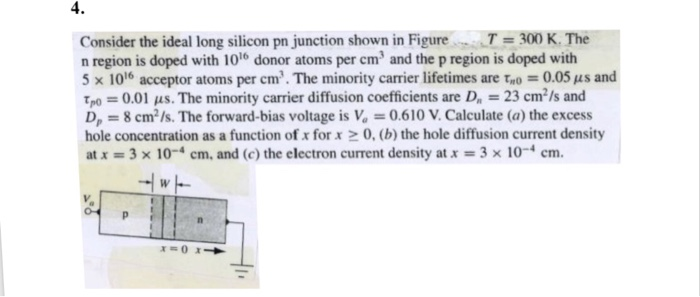3. A silicon step junction has uniform impurity doping concentrations of N. 5 x 1015 cm-3 and Nd = 1 x 1015 cm-, and a cross-sectional area of A-|0-4 cm2. Let tao -0.4 s and tpo 0.1 us. Consider the geometry in Figure.Calculate (a) the ideal reverse saturation current due to holes, (b) the ideal reverse saturation current due to electrons, (c) the hole concentration at a, if V V and (d) the electron current at x = x" + 1 LP for v, = .
4. Consider the ideal long silicon pn junction shown in FigureT300 K. The n region is doped with 100 donor atoms per cm3 and the p region is doped with 5 x 1016 acceptor atoms per cm. The minority carrier lifetimes are τ-o = 0.05 μ s and Tpo 0.01 us. The minority carrier diffusion coefficients are D 23 cm2/s and D, 8 cm2 /s. The forward-bias voltage is V 0.610 V. Calculate (a) the excess hole concentration as a function of x for x 0, (b) the hole diffusion current density at x 1-3 10-4 cm. and (c) the electron current density at x-3 x 10-4 cm.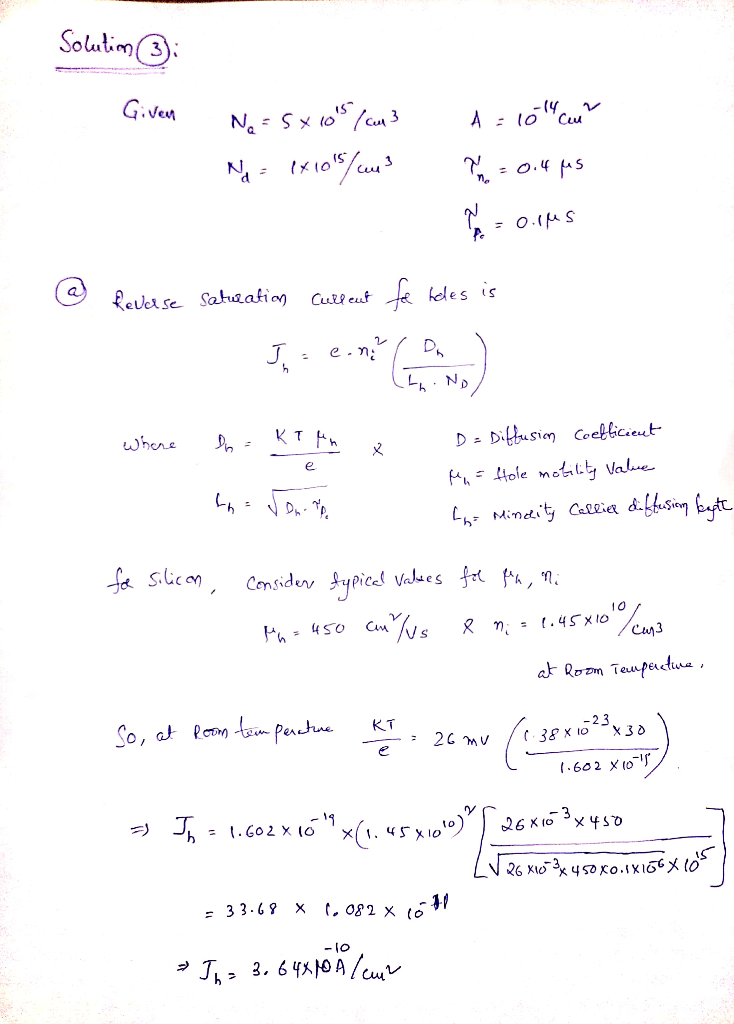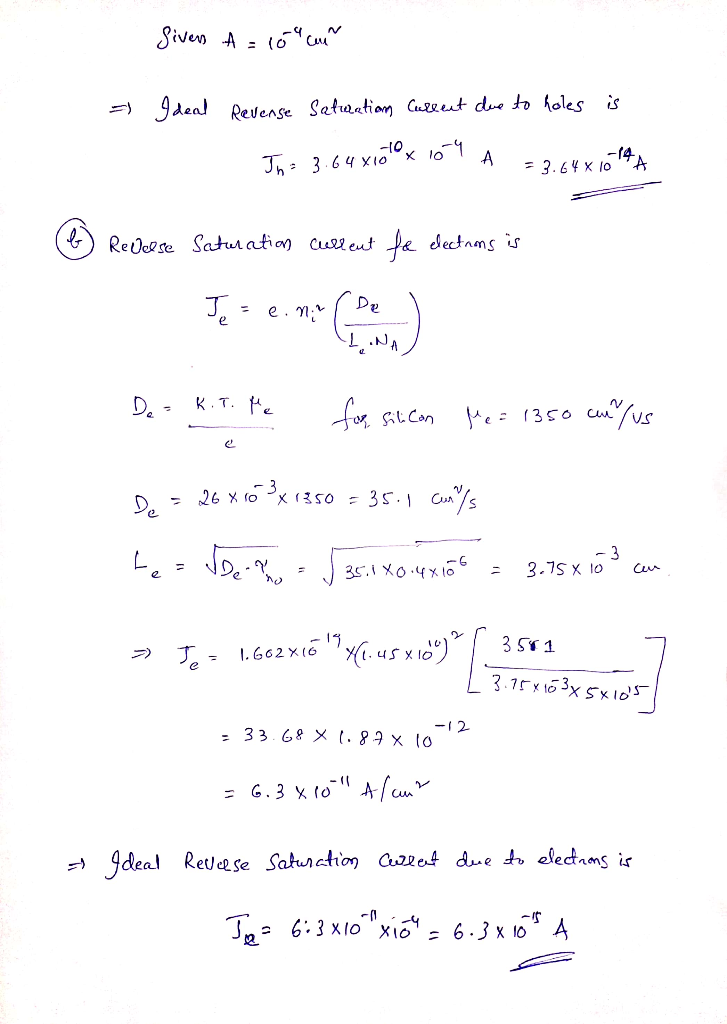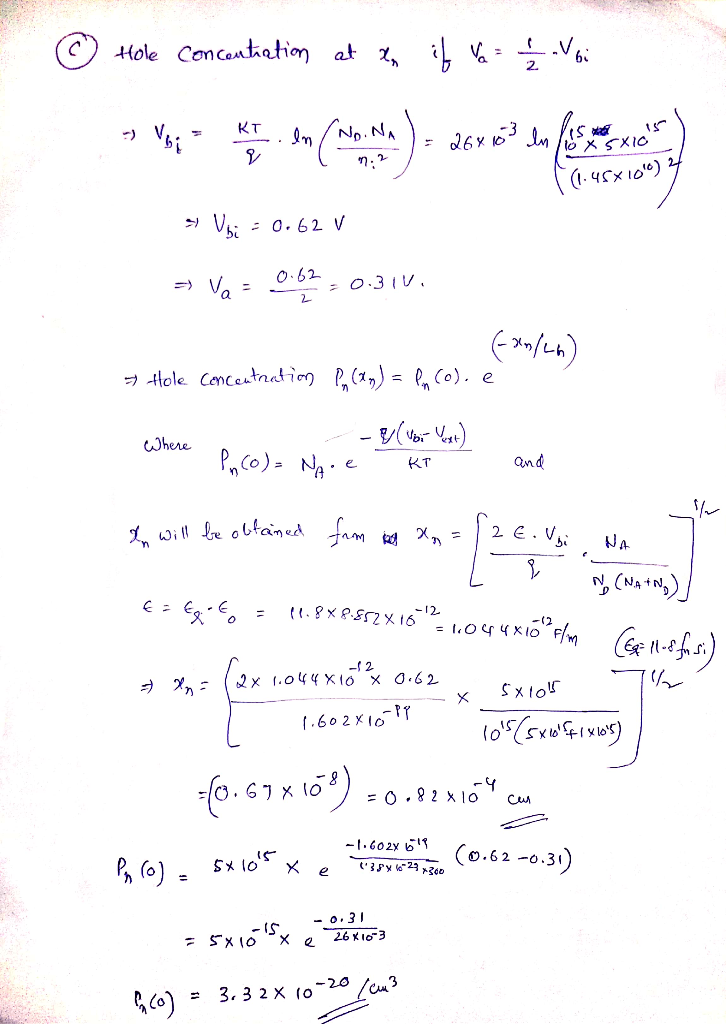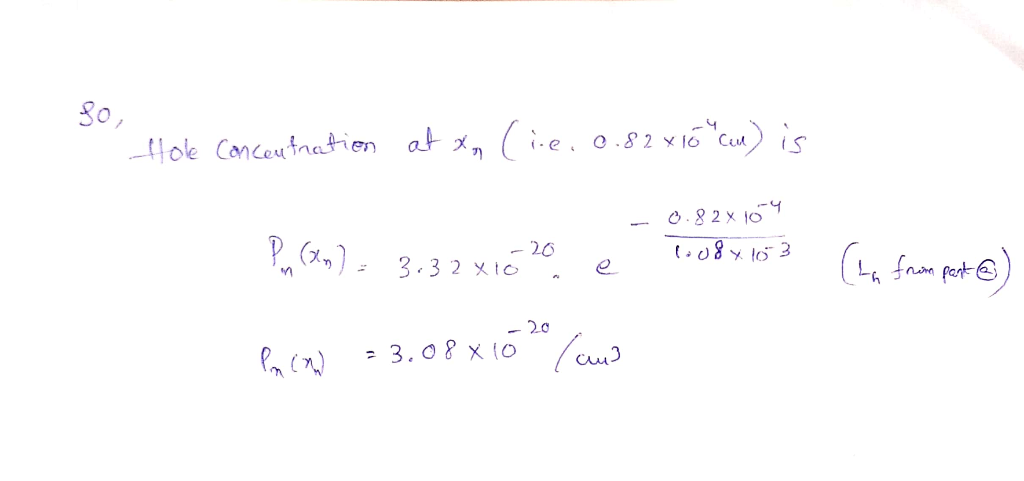##### Add Answer of: 3. A silicon step junction has uniform impurity doping concentrations of N. 5 x 1015 cm-3 and Nd ...
More Homework Help Questions Additional questions in this topic.

• #### will rate life saver Doping changes the Fermi energy of a semiconductor. Consider silicon, with...

Need Online Homework Help?